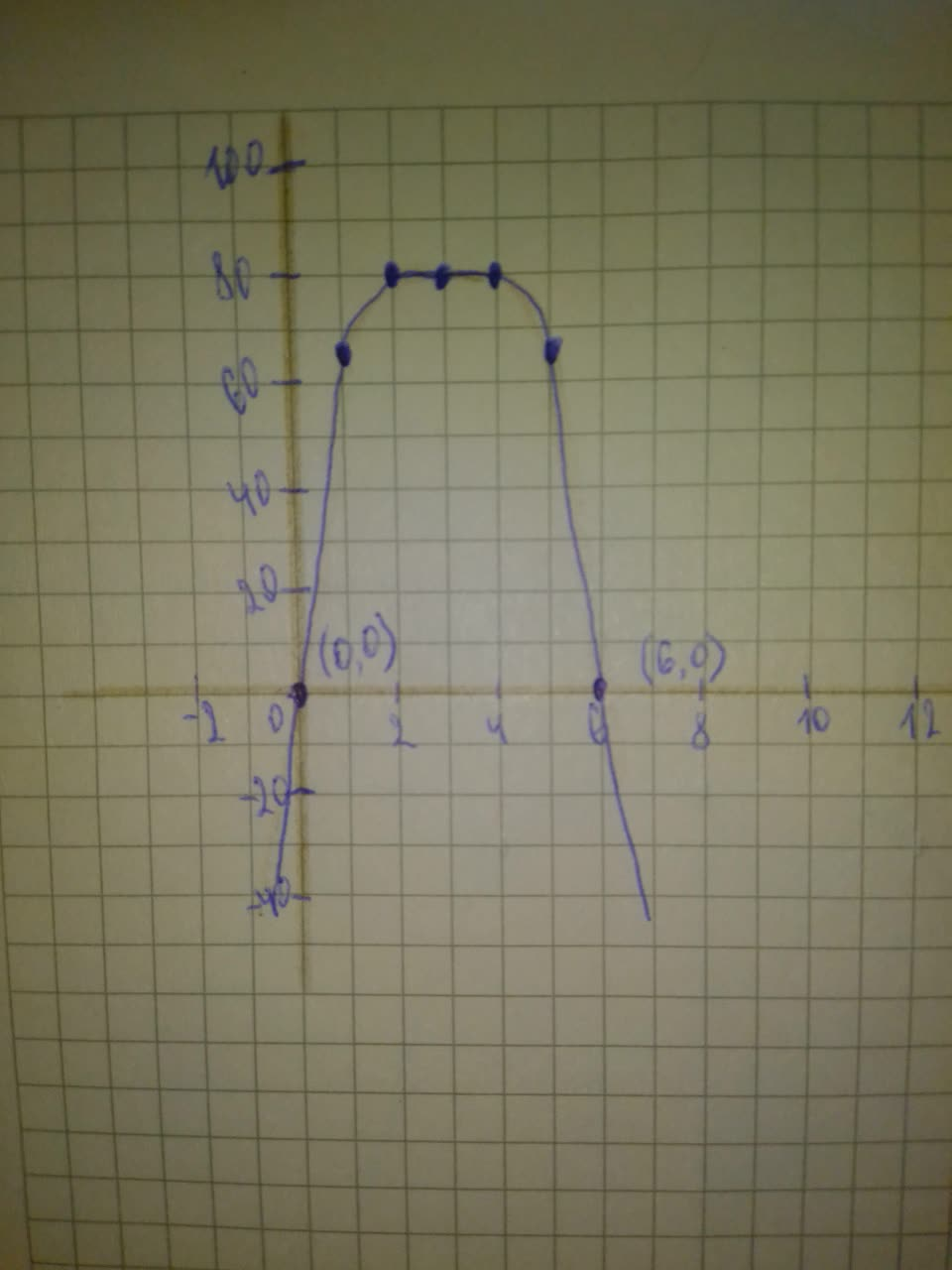Question# Graph the polynomial by transforming an appropriate graph of the form y=x^{n} and show clearly all x- and y-intercepts P(x)=81-(x-3)^{4}

Polynomial graphs
ANSWEREDGraph the polynomial by transforming an appropriate graph of the form $$\displaystyle{y}={x}^{{{n}}}$$.
Show clearly all x- and y-intercepts. $$\displaystyle{P}{\left({x}\right)}={81}-{\left({x}-{3}\right)}^{{{4}}}$$2021-08-02

Step 1
The parent function of $$\displaystyle{P}{\left({x}\right)}={81}-{\left({x}-{3}\right)}^{{{4}}}\ {i}{s}\ {y}={x}^{{{4}}}$$, which passed through the points $$\displaystyle{\left(-{2},{16}\right)},{\left(-{1},{1}\right)},{\left({0},{0}\right)},{\left({1},{1}\right)},{\quad\text{and}\quad}{\left({2},{16}\right)}.$$
$$\displaystyle{P}{\left({x}\right)}=-{\left({x}-{3}\right)}^{{{4}}}+{81}$$ is the graph of $$\displaystyle{y}={x}^{{{4}}}$$ reflected across the x-axis and then translated left 3 units right and up 81 units. Reflecting the points on $$\displaystyle{y}={x}^{{{4}}}$$ accross the x-axis given the points $$\displaystyle{\left(-{2},-{16}\right)},{\left(-{1},-{1}\right)},{\left({0},{0}\right)},{\left({1},-{1}\right)},{\quad\text{and}\quad}{\left({2},-{16}\right)}$$. Translating these points right 3 units and up 81 units then gives the points (1,65),(2,80),(3,81),(4,80), and (5,65).
The x-intercept of P(x) is when $$\displaystyle{P}{\left({x}\right)}={0}$$:
$$\displaystyle{P}{\left({x}\right)}={81}-{\left({x}-{3}\right)}^{{{4}}}$$ Given function.
$$\displaystyle{0}={81}-{\left({x}-{3}\right)}^{{{4}}}$$ Substitute $$\displaystyle{P}{\left({x}\right)}={0}$$
$$\displaystyle{\left({x}-{3}\right)}^{{{4}}}={81}$$ Add $$\displaystyle{\left({x}-{3}\right)}^{{{4}}}$$
$$\displaystyle{x}-{3}=\pm\sqrt{81}$$ Take the 4th root of both sides.
$$\displaystyle{x}-{3}=\pm{3}$$ Simplify
$$\displaystyle{x}={3}\pm{3}$$ Add 3 on both sides
The y-intercept of P(x) are then $$\displaystyle{x}={3}-{3}={0}{\quad\text{and}\quad}{x}={3}+{3}={6}$$
The y-intercept is when $$\displaystyle{x}={0}$$. From finding the x-intercept, we know $$\displaystyle{y}={0}$$ when $$\displaystyle{x}={0}$$ so the y-intercept is at (0,0).
Plot the points and then connect them with a smoth curve. Label the coordinates of the intercepts in your graph:Answer: See the explanation for the graph. To make the graph, reflect the graph of $$\displaystyle{y}={x}^{{{4}}}$$ across the x-axis and then translate right 3 units and up 81 units. Label the intercepts at (0,0) and (6,0)